Disclaimer: This is an example of a student written essay.

Any information contained within this essay is intended for educational purposes only. It should not be treated as authoritative or accurate when considering investments or other financial products.

# Fighting Credit Card Fraud

 ✅ Paper Type: Free Essay ✅ Subject: Finance ✅ Wordcount: 2963 words ✅ Published: 12th Oct 2021

## Introduction

As we become ever closer to a cashless society, the use of credit cards has become fundamental in everyday life. However, due to the increasing distribution of cards to UK residents, 158.9 million in 2018 rising to 159.2 million has given a proportional opportunity of credit card fraud. 

In the UK, fraud losses totalled to £620.6 million in 2019, with a total spend on all debit and credit cards reaching £829 billion in 2019 through 22 billion transactions made during the year. A key focus for banks and law enforcement is to prevent such fraud occurring as much as possible. Banks and card companies stopped £999.2 million of card fraud in 2019. This is equivalent to £6.17 in every £10 of attempted card fraud being prevented. 

If you need assistance with writing your essay, our professional essay writing service is here to help!

Within the Transactions Industry, fraud occurs through illegal usage of credit card details without the real cardholder's knowledge. Fraudulent transactions usually occur through counterfeit or stolen Credit Cards . Once a payment transaction has been spotted which the account holder did not make, they have the right to dispute the charge by contacting his/her bank. The bank or credit card company conducts an investigation (12). Within Mathematics lie an extensive list of credit card fraud detection methods such as Decision Trees, Genetic Algorithms & Neural Networks. (13)

To show a present time effect of credit card fraud being capitalised upon, through the COVID-19 pandemic, attempted fraudulent transactions rose by 35% in April 2020 in the United States. With an increase happening, there are numerous methods of tackling fraud - here we will be focusing on the use of Benford's Law.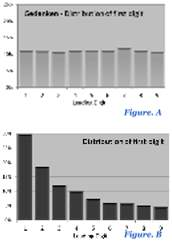## What is Benford's Law?

If I asked you how the occurrence of the first significant digits of a set of random numbers are distributed, most would feel (Fig. A) the Gendankan test portrays how it would be. However, the truth is that the lower the number, the more frequently it will occur (Fig, B). This is applicable to anything from numbers on the front page of the financial times, to the number of views of every video on Tik Tok; this is due to Benford's Law.

Benford's Law (The First Digit Law) states that the first significant digits are not uniformly distributed as expected. Instead they follow a logarithmic distribution asserting that the first significant digit (D1 ) does not have an equal probability of being one of nine possible digits 1, 2, …, 9. However 30% of the time, D​ ​1 will be 1, 17% of the time D​ ​1 will be 2, and this continues to D​ ​1 being 9 under 5% of the time with probabilities decreasing monotonically inbetween.

### How does Benford's Law Work?

This surprising law which applies to all aspects of life, is given from this equation of probability:

Pr(D1=d​ ​) = log10(1+ 1d ) for d​ ​∈ {1,2,...,9} (6)​

Here is the logarithmic equation for the probability of our first significant digit being D​1. Seeing this equation can be confusing as an explanation of why Pr(D​1 = 1) = 30.1%, Pr(D​1 = 2) = 17.6% and so on, this is down to the concept of Scale Invariance. (3)​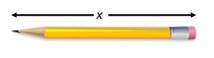Scale Invariance can be best visualised through a pencil with a length of one unit:

If we then increased the pencil's length, until we have doubled the length (increased 100%) it will be 1.xunits long until the first significant digit D 1 changes from 1 to 2.

​However, changing the length from D​ ​1= 2 to D​ ​1 = 3 the increase is only 50%. Best visualized on a logarithmic scale (Fig. C):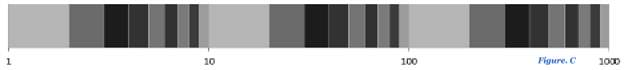This logarithmic scale shows moving along the scale, the distance between each subsequent mark becomes shorter until the next magnitude is achieved, showing the invariance of scale. Before a new magnitude, changing from D​1= 9 requires only an 11% change is required to change the value. However you can see the percentage of time that D1 = 1 is 30.1% of the time (the light grey area). (3)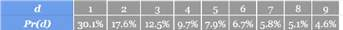Therefore, when finding the probability of the first significant digit d​ ​, it will be in the interval of

log(d + 1) − log(d) which gives us log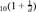-

our formula for the probability of D​ ​1. Inputting d​ ∈ {1,2,...,9} ​gives our probabilities:

Therefore for data to conform to Benford's Law it must be scale invariant. If we represent numbers in a scale invariant set of data as D1· 10k and multiply by a constant α which should not alter the distribution, where D1 is our first significant digit & 10k is the exponent. Giving us α · D1 · 10k , we can take logarithms: log(α · D1 · 10k) = log(α) + log(D1) + 10k which shows the uniform distribution on a logarithmic scale.

Scale Invariance is also proven through splitting the base 10 logarithm into their exponent and mantissa. Take x as a real positive number written in the form of x · y · 10n , where y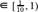is the mantissa & n ∈ℤ is the exponent (base 10), taking logs (base 10) giveslog(x) = log(y) + n , now let's use 3.45, log(3.45) = 0.537819 . (10)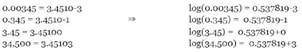As you can see, our mantissa (fractional part) repeats itself over all magnitudes, the first significant digit (D1 ) of our mantissa in this example is 5. If we plotted D1 of the mantissa of a common logarithm against a logarithmic scale - we have: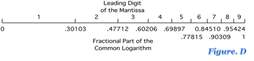As you can see, the distribution results in D1 equalling 1 having the largest interval over the interval of D1 = 2, 3, …, 9.

### Does it apply for the Second Digit?

If a distribution for the first digit exists, this would naturally lead to a distribution for the second digit existing. There is in fact a distribution for any digit. So the probability, for example, of the second digit being a 4 is just the sum of the probabilities of the number being 1.4, 2.4, 3.4 … all the way up to 9.3. (4)

This is stated as the joint distribution – the probability of the second digit having a particular value is given that the first digit has another value thus this probability not being independent. Therefore giving us the General Significant Digit Law:

−1 k

P(D1 = d1,...,Dk = dk) = log10(1 + (∑ di10ki) for d1 = 0...9 , kN ,dj = 0...9 ,j = 2...k (6)

i=1

For example, if digits follow the Benford distribution, the combination of significant digits 1129 (e.g. 0.001129) is expected with probability log10[1 +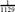] . This "general significant-digit law"allows the occurrence of the marginal distributions of second-order and higher-order digits. (4)

## So how can Benford's Law be used in Finance?

Within foreign exchange & stock pricing, converting from Dollars Per stock to Pesos Per Stock will retain similar first digit frequencies irrespective of the first digit of the stock prices changing radically. This is also apparent if changing stock pricing from Dollars Per Stock to Stocks Per Dollar, however if we applied this to stock tables not close to Benford's Law (such as uniformly distributed prices), we would see a clear change in D1 frequencies. (6)

Purchases can range from negligible penny transactions amounts to mortgage repayments all the way to buying homes outright. Due to scale invariance these transactions would span several magnitudes when expressed as α - D1 - 10k therefore creating a broad probability distribution that would make it possible for financial & Credit Card Statements to conform to Benford's Law.

Benford's Law is used extensively in the testing of fabricated data, only applicable to data that spreads over large magnitudes - which is shown by the proof through a logarithmic scale. We can look at two sets of data which satisfy the conditions for being tested against Benford's Law:

## Putting Benford's Law To The Test

Due to the strength and versatility of Benford's Law, we have seen that financial data can span magnitudes and obey scale invariance. Therefore we are able to analyse two examples of credit card data: fraudulent and non-fraudulent. Using Benford's Law to detect fraudulent data, we will analyse a plot against Benford's Law and then a statistical to find the goodness-of-fit to Benford's Distribution to test against our null and alternative hypotheses. Using Google Sheets we are able to compute the given value for the following tests using the LEFT and COUNTIF functions to find D1 of each value and sum up the number of observations. (7) Statistical Test: Chi-Squared Goodness-of-fit

Due to looking into if data is compliant with Benford's Distribution, the chi - squared goodness-of-fit is the ideal hypothesis test. ​ We​ will calculate our chi - squared statistic using this formula:

9 2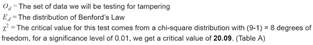χ2 =(Od EdEd)

d=1

Producing our null & alternative hypotheses:

H0 : χ2 ≤ 20.09- The first significant digits in this set of data obey Benford's Law.

H1 : χ2 > 20.09- The first significant digits in this set of data do not obey Benford's Law.

For each example, through Google Sheets we have computed each step of our chi-squared hypothesis test, finding the frequency of each first significant digit, the expected occurrence of this digit in relation to Benford's distribution, finally plugging our values into our chi-squared formula. Finally summing up our final column to give our critical value:

### 1) State of Arizona v. Wayne James Nelson (CV92-18841)

In 1993, in State of Arizona v. Wayne James Nelson (CV92-18841), the accused was found guilty of trying to defraud the state of nearly \$2 million, by diverting funds to a bogus vendor. (8)

Graphical & Numerical Analysis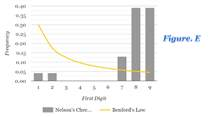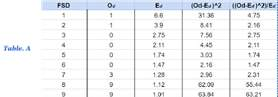Here the first significant digits being 1 and 9 at 5% and 38% respectively, there is also a clear gap of 0% for first significant digits being 3 to 6. This clearly does not conform to Benford's Law due to no correlation. The data does span magnitudes and expresses scale invariance, however the fraudulent values do not conform to Benford's Law. (Fig E)

Statistical Test

χ2 = 4.75 + 2.16 + ... + 55.44 = 135.94

As χ2 > 20.09, we are able to reject H 0​ and accept H ​1 Therefore sufficient​ evidence that this data does not conform to Benford's Law and may be subjected to tampering.

### 2) Credit Card Users v Expenditure Per User

Here we have a set of 187 Credit Card users and their expenditure over a set period of time. Due to being scale invariant, spanning magnitudes and randomly collected - we expect this set of data to conform to Benford's Law. (9)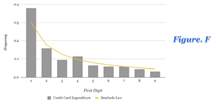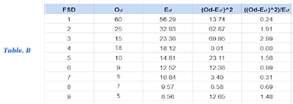Graphical & Numerical Analysis

In this set of data, it is clear that the first significant digit equalling 1 occurs more frequently than all other digits. Like Benford's Law, the occurrence of each first significant digit, decreases as D​ ​1​increases. (Fig F)

Statistical Test

χ2 = 0.24 + 1.91 + ... + 1.48 = 10.18

As χ2 < 20.09, there is sufficient evidence to accept H 0​ thus rejecting H 1​ , proving that this data conforms to Benford's Law.

## Overall Thoughts & Conclusion

As we have seen, data that does meet the given requirements of testing against Benford's Law will give a clear indication of the validity of the data we are looking at. However, financial data will not always meet the requirements such as spanning numerous magnitudes; nor will this law help spot which values are fraudulent, but instead the presence. For this reason Benford's Law must not be used as a primary test to determine the validity of Financial Data, but should be used more as a supporting test. We can also use our Chi - Squared tested in correspondence with the further digits using the general significant digit distribution we looked at earlier, thus giving a more effective use of Benford's Law in testing for the presence of fraudulent data.

## References

(1) Fraud, the Facts - UK Finance 2020: https://www.ukfinance.org.uk/policy-and-guidance/reports-publications/fraud-facts-2020

(2) 15 Disturbing Credit Card Facts 2021:

(3) Data Genetics: Benford's Law: https://www.datagenetics.com/blog/march52012/index.html

(4) Second Digit Phenomenon:

https://econwpa.ub.uni-muenchen.de/econ-wp/othr/papers/0507/0507001.pdf

(5) Barrow, P. J. (2011, February Tuesday). Benford's Very Strange Law. Retrieved from Gresham College:

www.gresham.ac.uk/lectures-and-events/benfords-very-strange-law

(6) Encyclopedia of Mathematics: Benford's Law:

https://encyclopediaofmath.org/wiki/Benford_law

(7) Detecting Numeric Irregularities with Benford's Law:

https://blog.bigml.com/2015/05/15/detecting-numeric-irregularities-with-benfords-law/

(8) How to Commit Tax Fraud: http://alfre.dk/how-to-commit-tax-fraud/

(9) Credit Card Client Data:

https://www.kaggle.com/mariosfish/default-of-credit-card-clients

(10) Stack Exchange - How does Benford's Law Work, Rob John, January

2013:https://math.stackexchange.com/questions/781/why-does-benfords-law-or-zipfs-law-hold/291662#291662

(11) Hills, T. (1998). The First Digit Phenomenon. American Scientist 86,:

https://hill.math.gatech.edu/publications/PAPER%20PDFS/TheFirstDigitPhenomenonAmericanScientist1996.pdf

(12) What is a Fraudulent Transaction? - Cardinality:

https://cardinity.com/faq/what-is-a-fraudulent-transaction

(13) http://usir.salford.ac.uk/id/eprint/2595/1/BBS.pdf - Credit card fraud and detection techniques : a review (Delamaire, L, Abdou, HAH and Pointon, J, 2009)

## Figures

(A) https://www.datagenetics.com/blog/march52012/index.html (B) https://www.datagenetics.com/blog/march52012/index.html

(F) http://alfre.dk/how-to-commit-tax-fraud/

View all

## DMCA / Removal Request

If you are the original writer of this essay and no longer wish to have your work published on UKEssays.com then please:

Related Services

Prices from

KD47

Approximate costs for:

• 1000 words
• 7 day deliveryHumanity University

Dedicated to your worth and value as a human being!

Related Lectures